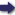(London :  Kegan Paul, Trench, Trübner & Co.,  1910.)

 Tools

## Search this bookPrev Page 39 Next``` CHAPTER LII. 39 till the moment of the gauge-date are 1,972,948,132. The latter We write down this number in three different places, piled to the The upper number we multiply by ten, by which it gets a cipher more at the right side. The middle number we multiply by 2481 and get the product 4,894,884,315,492. The lower number v/e multiply by Page 222. 7739, and get the product 15,268,645,593,548. The latter two numbers we divide by 9600; thereby we get for the middle number as quotient 509,883,782 and a remainder of 8292, and for the lower number a quo¬ tient of 1,590,483,915 and a remainder of 9548. The sum of these two remainders is 17,840. This fraction (i.e. ^l-g-Vjf) is reckoned as one whole. Thereby the sum of the numbers in all three places is raised to 21,829,849,018, i.e. ctdhimdsa days, plus ^-f-g- day of the current adhimdsa day (i.e. which is now in course of summing up). Eeducing these days to months, we get 727,661,633 months and a remainder of twenty-eight days, which is called Sh-D-D. This is the interval between the beginning of the month Caitra, which is not omitted in the series of months, and the moment of the vernal equinox. Further, adding the quotient which we have got for the middle number to the years of the kalpa, we get the sum of 2,482,831,914. Dividing this number by 7, we get the remainder 3. Therefore the sun has, in the year in question, entered Aries on a Tuesday. The two numbers which are used as multiplicators Explanatory for the numbers in the middle and lower places are to latter be explained in the following manner:— Dividing the civil days of a kcdpa by the solar cycles of a kalpa, we get as quotient the number of days which compose a year, i.e. Z^S\',^^%\-^^%\%%^' Eeducing this fraction by the common divisor of 450,000, we get 365f|-7ro'- The fraction may be further reduced by being divided by 3, but people leave it as it is, in order ```Prev Page 39 Next